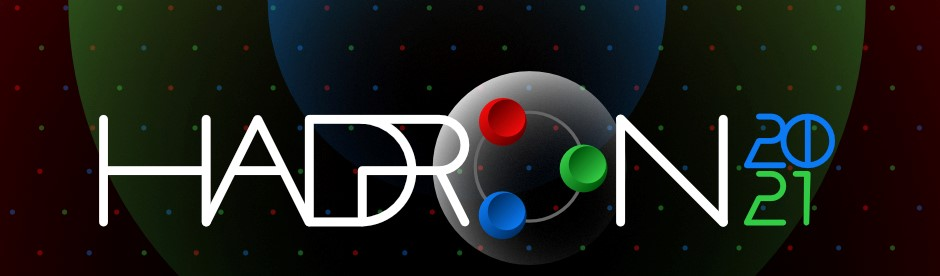#19th International Conference on Hadron Spectroscopy and Structure in memoriam Simon Eidelman

26-31 July 2021
Mexico City
Mexico/General timezone
HADRON 2021 is over. Thanks for making it a success!
Home > Timetable > Session details
PDF | iCal

## Place

Location: Mexico City
Date: 29 Jul 11:15 - 13:15

## Conveners

• Dr. Pilloni, Alessandro (Università di Messina)

## Timetable | Contribution List

Displaying 5 contributions out of 5
Type: Parallel Session: Exotic hadrons and candidates-6
In this talk, I will provide distinguishable consequences of the $Z_{cs}(3985)$ and $Z_{cs}(4000)$ as two or one state. In the one-state scheme, $Z_{cs}(3985/4000)^−$ state is the U-spin partner of $Z_c(3900)^−$. Otherwise, the SU(3) flavor partner of $Z_{c}(3900)$ is $Z_{cs}(4000)$ rather than the $Z_{cs}(3985)$. Another two important predictions in the two-state scheme are the existence of a ... More
Presented by Dr. Lu MENG on 29/7/2021 at 12:00
Type: Parallel Session: Exotic hadrons and candidates-6
We have recently studied the interactions of $D-\bar D + c.c$ with a nucleon, with the motivation to find formation of $P_c$ like states. We consider all interactions in s-wave and solve scattering equations. We find formation of nucleons with hidden charm, spin 1/2, 3/2 and positive parity. We earlier studied the interaction of $D-\bar D$ with a kaon, where a $K^*$-meson is found to arise. In th ... More
Presented by Ms. Brenda MALABARBA on 29/7/2021 at 12:40
We present a recent investigation on the production of $P_{cs}(4459)$ in the exclusive $K^-p\to J/\psi\Lambda$ reaction using both the effective Lagrangian and Regge approaches. We assume six different spin and parity assignments, i.e., $1/2^\pm$, $3/2^\pm$ and $5/2^\pm$ to the newly found $P_{cs}$. The total and differential cross sections for the $K^-p\to J/\psi\Lambda$ reaction are calculated. ... More
We have studied the $e^+ e^- \to K^+ ( D_s^{*-} D^0 + D_s^- D^{*0})$ reaction recently measured at BESIII, from where a new exotic $Z_{cs}$ state has been reported. We study the interaction of $\bar D_s D^*$ with the coupled channels. Although our picture does not produce a bound state nor resonance, the interaction of the coupled channels has sufficient strength to produce the enhancement clo ... More